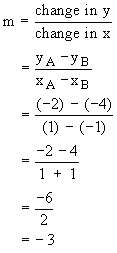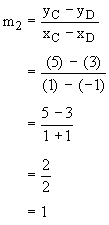Home MonkeyNotes Printable Notes Digital Library Study Guides Study Smart Parents Tips College Planning Test Prep Fun Zone Help / FAQ How to Cite New Title Request

 Example Find the slope of the graph y = -3x + 1 using coordinates. Solution : y = -3x + 1, put x = 1, then y = -3(1) + 1 = -2 Let A(1, -2) and put x = -1 then y = -3 (-1) +1 = 4 Let B (-1, 4) Pick these two points A(1,-2) and B(-1,4) and calculate the slope 'm'Example Are the two lines y + x = 2 and y = x + 4 parallel? Solution : y + x = 2 put x = 1 then y = 1. Let A(1,1) put x = -1 then y = 3. Let B (-1, 3) Therefore, the slope of line AB i.e. y + x = 2 isAlso y = x + 4 put x =1 then y = 5 Let C (1,5) and put x = -1 then y = 3 Let D (-1,3) Therefore, slope of line CD i.e. y = x + 4 isSince the two slopes m1 and m2 are not equal the two lines are not parallel to each other. Index 8.1 Definition8.2 Relations, Graphs and Symmetry8.3 Slopes and Intercepts Chapter 9
 Search: All Products Books Popular Music Classical Music Video DVD Toys & Games Electronics Software Tools & Hardware Outdoor Living Kitchen & Housewares Camera & Photo Cell Phones Keywords: